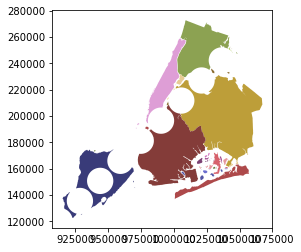Spatial overlays allow you to compare two GeoDataFrames containing polygon or multipolygon geometries and create a new GeoDataFrame with the new geometries representing the spatial combination and merged properties. This allows you to answer questions like

What are the demographics of the census tracts within 1000 ft of the highway?

The basic idea is demonstrated by the graphic below but keep in mind that overlays operate at the dataframe level, not on individual geometries, and the properties from both are retained

In :
from IPython.core.display import Image
Image(url="http://docs.qgis.org/testing/en/_images/overlay_operations.png")

Out: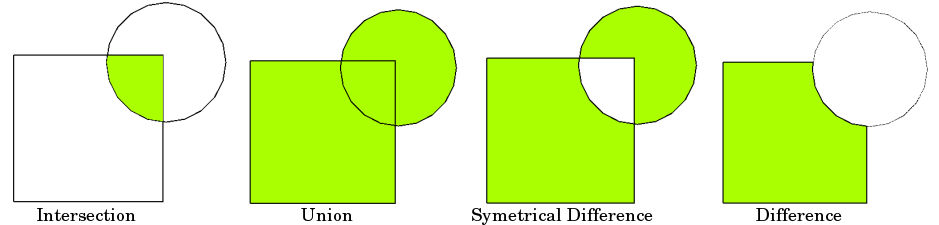Now we can load up two GeoDataFrames containing (multi)polygon geometries...

In :
%matplotlib inline
from shapely.geometry import Point
from geopandas import datasets, GeoDataFrame, read_file
from geopandas.tools import overlay

# NYC Boros
zippath = datasets.get_path('nybb')
polydf = read_file(zippath)

# Generate some circles
b = [int(x) for x in polydf.total_bounds]
N = 10
polydf2 = GeoDataFrame([
{'geometry': Point(x, y).buffer(10000), 'value1': x + y, 'value2': x - y}
for x, y in zip(range(b, b, int((b - b) / N)),
range(b, b, int((b - b) / N)))])


The first dataframe contains multipolygons of the NYC boros

In :
polydf.plot()

Out:
<matplotlib.axes._subplots.AxesSubplot at 0x512ee48>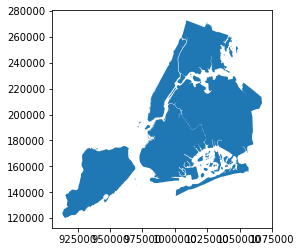And the second GeoDataFrame is a sequentially generated set of circles in the same geographic space. We'll plot these with a different color palette.

In :
polydf2.plot(cmap='tab20b')

Out:
<matplotlib.axes._subplots.AxesSubplot at 0x512e0f0>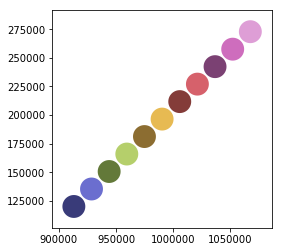The geopandas.tools.overlay function takes three arguments:

• df1
• df2
• how

Where how can be one of:

['intersection',
'union',
'identity',
'symmetric_difference',
'difference']



So let's identify the areas (and attributes) where both dataframes intersect using the overlay tool.

In :
from geopandas.tools import overlay
newdf = overlay(polydf, polydf2, how="intersection")
newdf.plot(cmap='tab20b')

Out:
<matplotlib.axes._subplots.AxesSubplot at 0x12978940>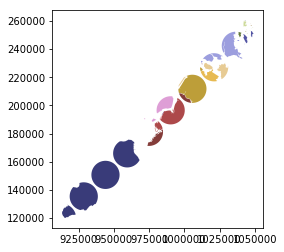And take a look at the attributes; we see that the attributes from both of the original GeoDataFrames are retained.

In :
polydf.head()

Out:
BoroCode BoroName Shape_Leng Shape_Area geometry
0 5 Staten Island 330470.010332 1.623820e+09 (POLYGON ((970217.0223999023 145643.3322143555...
1 4 Queens 896344.047763 3.045213e+09 (POLYGON ((1029606.076599121 156073.8142089844...
2 3 Brooklyn 741080.523166 1.937479e+09 (POLYGON ((1021176.479003906 151374.7969970703...
3 1 Manhattan 359299.096471 6.364715e+08 (POLYGON ((981219.0557861328 188655.3157958984...
4 2 Bronx 464392.991824 1.186925e+09 (POLYGON ((1012821.805786133 229228.2645874023...
In :
polydf2.head()

Out:
geometry value1 value2
0 POLYGON ((923175 120121, 923126.847266722 1191... 1033296 793054
1 POLYGON ((938595 135393, 938546.847266722 1344... 1063988 793202
2 POLYGON ((954015 150665, 953966.847266722 1496... 1094680 793350
3 POLYGON ((969435 165937, 969386.847266722 1649... 1125372 793498
4 POLYGON ((984855 181209, 984806.847266722 1802... 1156064 793646
In :
newdf.head()

Out:
BoroCode BoroName Shape_Leng Shape_Area value1 value2 geometry
0 5 Staten Island 330470.010332 1.623820e+09 1033296 793054 POLYGON ((916755.4256330276 129447.9617643995,...
1 5 Staten Island 330470.010332 1.623820e+09 1063988 793202 POLYGON ((938595 135393, 938546.847266722 1344...
2 5 Staten Island 330470.010332 1.623820e+09 1125372 793498 POLYGON ((961436.3049926758 175473.0296020508,...
3 5 Staten Island 330470.010332 1.623820e+09 1094680 793350 POLYGON ((954015 150665, 953966.847266722 1496...
4 2 Bronx 464392.991824 1.186925e+09 1309524 794386 POLYGON ((1043287.193237305 260300.0289916992,...

Now let's look at the other how operations:

In :
newdf = overlay(polydf, polydf2, how="union")
newdf.plot(cmap='tab20b')

Out:
<matplotlib.axes._subplots.AxesSubplot at 0x12a72e48>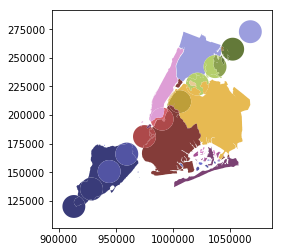In :
newdf = overlay(polydf, polydf2, how="identity")
newdf.plot(cmap='tab20b')

Out:
<matplotlib.axes._subplots.AxesSubplot at 0x12bccda0>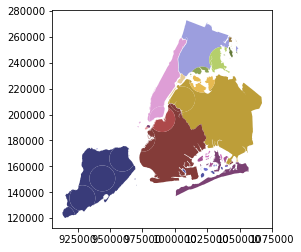In :
newdf = overlay(polydf, polydf2, how="symmetric_difference")
newdf.plot(cmap='tab20b')

Out:
<matplotlib.axes._subplots.AxesSubplot at 0x12dc2710>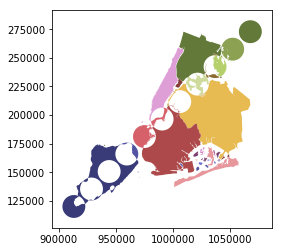In :
newdf = overlay(polydf, polydf2, how="difference")
newdf.plot(cmap='tab20b')

Out:
<matplotlib.axes._subplots.AxesSubplot at 0x12e13630>